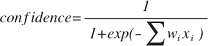# 1.0 - 8.00 - SparseSVMPredictor Output - Teradata Vantage

## Teradata® Vantage Machine Learning Engine Analytic Function Reference

Product
Release Number
1.0
8.00
Release Date
May 2019
Content Type
Programming Reference
Publication ID
B700-4003-098K
Language
English (United States)

## Output Table Schema

The table has the predicted class of each test sample.

Column Data Type Description
id_column INTEGER, SMALLINT, BIGINT, NUMERIC, VARCHAR, or VARBYTE(n) Test sample identifier.
predict_value VARCHAR Predicted class of test sample.
predict_confidence DOUBLE PRECISION Prediction confidence, a value between 0 and 1, computed by formula that follows this table. The higher the value, the more dependable the prediction.
accumulate_column Any [Column appears once for each specified accumulate_column.] Column copied from input table.

## Formula for predict_confidencewhere i is the attribute id, x i is the value of attributes in the sample, and w i is the weight of attribute i.